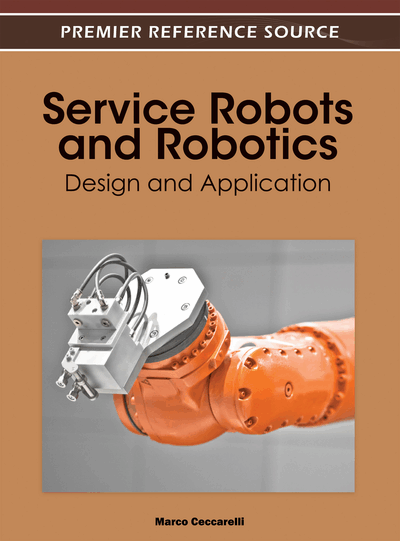# Kinematics Design for Structures and Algorithms

Ding Xilun (Beijing University of Aeronautics and Astronautics, China), Chen Hao (Beijing University of Aeronautics and Astronautics, China) and Xu Kun (Beijing University of Aeronautics and Astronautics, China)
Copyright: © 2012 |Pages: 15
DOI: 10.4018/978-1-4666-0291-5.ch002
OnDemand PDF Download:
Available
\$37.50
No Current Special Offers

## Abstract

The purpose of this chapter is to present the kinematic analysis of service robots using the Product of Exponential (PoE) formula. The forward kinematics problem and inverse kinematics problem are discussed and the Jacobian is obtained too. A model of quadruped robot will be used as an example in corresponding sections in order to facilitate understanding.
Chapter Preview
Top

## Introduction

In recent decades, the development of robot applications is constantly expanding with a very important tendency that robots have gradually shifted from manufacturing to non-manufacturing and service industries. Service industries include cleaning, oiling, ambulance, rescue, relief, etc. Compared with industrial robots, service robots have an apparent difference that they are generally a mobile platform, which can carry some manipulators on board, as well as with a number of haptic sensors and vision sensors, ultrasonic distance sensors, and so on. In this sense, the kinematics of service robots is similar with the kinematics of mobile robots.

In this chapter, we discuss the kinematics problems for service robots. Kinematics is the most fundamental aspect of robot design, analysis, control, and simulation. This chapter is constituted of 4 sections, and each section illustrates one topic of kinematics. We will present the representations of rigid body motion, the forward and inverse kinematics, as well as Jacobians. In order to facilitate understanding, a model of quadruped robot will be used as an example in corresponding sections. Our purpose is to introduce an overall idea of kinematics problems and relative topics to readers and to provide guidance in analysis and design of real robots.

In Figure 1 is the kinematic sketch of our quadruped robot at Beijing University of Aeronautics and Astronautics (Ding & Xu, 2009). Its leg can be seen as a serial mechanism, while the entire body can be seen as a parallel mechanism with three or four serial chains. Consequently, it is an appropriate example for kinematics analysis.

Top

## Rigid Body Motion

### Homogeneous Transformations

A robot is generally constituted of rigid bodies and joints, so this chapter begins with the study of rigid body motion. A rigid motion of an object is a motion, which preserves distance between points. The study on rigid body motion is the foundation of robotic kinematics. We are going to give some description of the motion of rigid bodies.

To facilitate illustration, two coordinate reference frames are established here. S is an inertial frame, while T is a movable frame attached to a rigid body. Assume that the T frame moves relative to the S frame.

Generally speaking, rigid motions are constituted of rotation and translation. The translational motion is simply represented by a 3×1 vector

(1)

The components of this vector are the Cartesian coordinates of origin of the S frame, which are the projections of the vector pST onto the corresponding axes.

The rotational motion is represented by a 3×3 matrix

(2)

RST is called a rotation matrix of which the components are the dot products of basis vectors of the two coordinate frames.

A rotation matrix has two key properties that follow from its construction. Letbe a rotation matrix, we have:

The set of all 3×3 matrices which satisfy these two properties is denoted as SO(3) (for special orthogonal group):

SO(3) is a group using the identity matrix I as the identity element and matrix multiplication as the group operation (Hervé, 1999). SO(3) represents the rotation group of.

A configuration of the system consists of the pair (pST, RST), and the configuration space of the system is the product space ofwith SO(3), which shall be denoted as SE(3) (for special Euclidean group):

An element (p,R)SE(3) serves as both a specification of the configuration of a rigid body and a transformation taking the coordinates of a point from one frame to another. Any vector rT expressed in the T coordinate frame can be expressed in the S coordinate frame if the position and orientation of the T frame are known relative to the S frame. Thus:

rS = pST + RSTrT(3)

This equation can be written as:

(4) where: (5) is the homogeneous representation of gST = (pST, RST)SE(3), [rS, 1]T and [rT, 1]T are the homogeneous representations of the position vectors rS and rT respectively.

## Complete Chapter List

Search this Book:
Reset